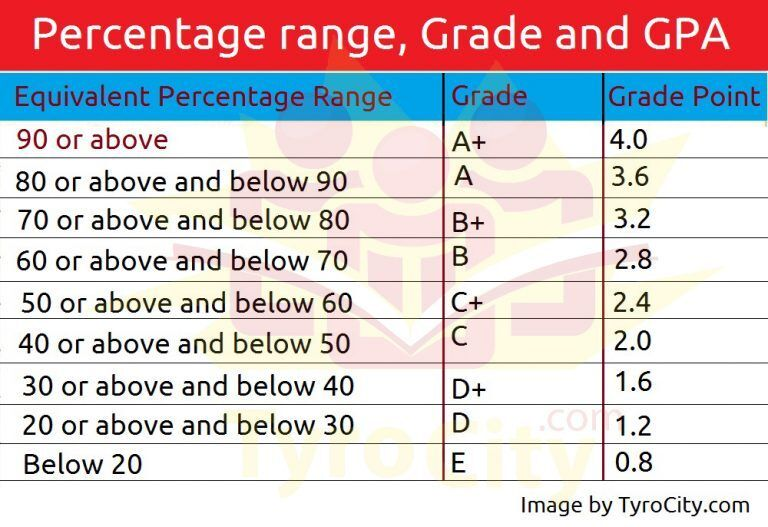## TyroCity

Tyro News for News

Posted on • Updated on

# Explaining GPA (SLC result)

Wondering what actually is difference or relation between percentage, GPA, grade points?

GPA or Grade Point Average is calculated by taking the number of grade points a student earned in a given period of time divided by the total number of credits taken (4, here). For every subject a total of 128 clock hrs. is the time that a student must be studying the subject. SLC Board considers that a credit hour will include a total of 32 clock hours. Now, with simple mathematics, we get 4 credit hour which is the base max value for GPA as well.

For example, if a student earns a grade of “A+”, he or she would have a Grade Point Average of 3.6 out of 4 (for Excellent or Superior Performance).

Here is a full list of it:Remember, GPA gives a range of marks you got not exact marks and is considered more standard way of grading. It is in no way related to percentage. Any attempt to calculate the percentage with the use of GPA is useless and hold no value.